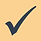top of page

## Topic 7: Negative integersNegative integers are integers with a value less than zero.

A number line is a good way to visualise negative integers.

Most Level 1 exam questions on negative integers can be answered by using a number line like this, simply by moving up or down the line.

Here are some examples.

3 – 8 = -5

-4 + 10 = 6

-1 – 6 = -7

-7 + 5 = -2###### Positive integersbottom of page